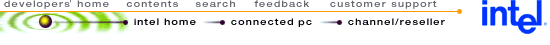## Using MMX™ Instructions to Implement Viterbi Decoding

 Disclaimer Information in this document is provided in connection with Intel products. No license, express or implied, by estoppel or otherwise, to any intellectual property rights is granted by this document. Except as provided in Intel's Terms and Conditions of Sale for such products, Intel assumes no liability whatsoever, and Intel disclaims any express or implied warranty, relating to sale and/or use of Intel products including liability or warranties relating to fitness for a particular purpose, merchantability, or infringement of any patent, copyright or other intellectual property right. Intel products are not intended for use in medical, life saving, or life sustaining applications. Intel may make changes to specifications and product descriptions at any time, without notice. Copyright © Intel Corporation (1996). Third-party brands and names are the property of their respective owners.

 1.0. INTRODUCTION 1.1. The Viterbi Decoding Algorithm 1.2. HMM References2.1. Core of viterbi_mmx 2.2. Finding the Minimum 2.3. Alignment of Operands

## 1.0. INTRODUCTION

The Intel Architecture (IA) media extensions include single-instruction, multi-data (SIMD) instructions. This application note presents a code example that implements the Viterbi decoding algorithm. These extensions include single-instruction multiple-data (SIMD) instructions that can operate in parallel on eight-byte (8-bit) operands, four-word (16-bit) operands or two long (32-bit) operands. Using these instructions, the Viterbi decoding algorithm shows a performance gain of 2X, over normal IA (scalar) code, because the data is manipulated 64-bits at a time. In this implementation, 32-bit operands are used, therefore two such operands can be manipulated (add, subtract etc.) in parallel in a single clock cycle.

### 1.1. The Viterbi Decoding Algorithm

In this section a very brief description of Hidden Markov Models (HMM) and the Viterbi algorithm is given. The reader is encouraged to consider the references listed in Section 1.2. for a more detailed coverage of this topic and its relationship to speech recognition.

Viterbi decoding is a Dynamic Programming (DP) algorithm that, among other applications, is used in evaluating Hidden Markov Models. An HMM consists of N states where transitions can occur from one state i to another state j with a probability a(j,i) called the transition probability. The probability of being in state i at time t = 1 is p(i) and is called the initial probability. When the constraint a(j,i) = 0 for j > i + 2 applies, then the HMM is known as a constrained-jump model and is frequently used for speech recognition. The connectivity of such a model is shown in Figure 1. Note the transitions from state 1 to states 1, 2, and 3 but not to 4.

Figure 1. Hidden Markov Model (Constrained-Jump)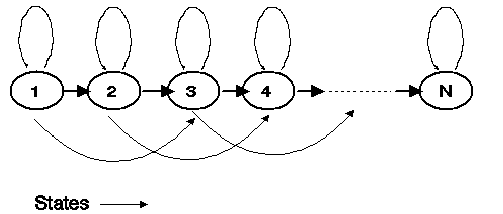When modeling speech on a transition occurring to state j at time t, a continuous output observation O(t) (usually a speech feature vector) is generated with a probability b(i,O(t)) called the output probability. In a discrete HMM, the continuous observation vector O(t) is Vector Quantisized (VQ) to a single discrete VQ index k(t) that is then used to lookup the output probability b(i,k(t)). Frequently, this is written in short form as b(i, t).

Thus, given an observation sequence O(t), t = 1..T, one can compute the probability that the observation sequence can be generated by a given HMM. There will be many paths (i.e. state sequences) in the HMM that can generate the same observation sequence - however, with different probabilities.

The Viterbi decoding algorithm computes the probability of the best (highest probability) path including (optionally) the sequence of states in the best path. This probability is known as the Viterbi probability PV which can be computed for an observation sequence O(t), t = 1,..,T as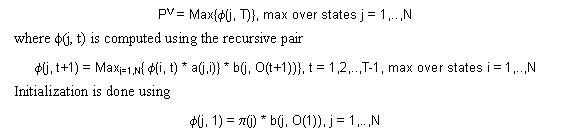In this implementation, negative log probabilities (base 10) are used (so we end up with all positive numbers) because then the multiplication changes to an addition, mitigating the scaling issues. Furthermore, only the constrained-jump model discrete HMM is assumed. Therefore, taking negative logarithms on each side the above three equations reduce to: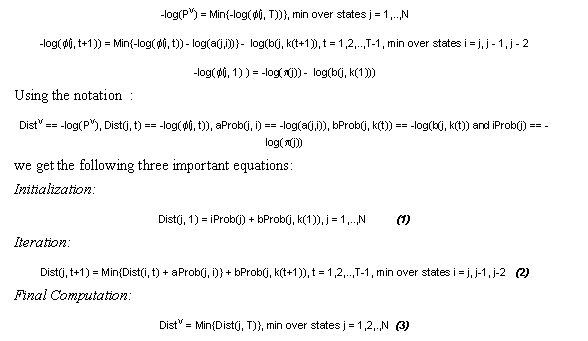Sometimes it might be desirable to store the best path sequence also in addition to the best path distance. In this implementation only the best path distance is computed.

Figure 2. Viterbi LatticeFigure 2. shows the lattice that is used to compute equation (2). At each time index this computation is done for all states (i.e. for j = 1 to N).

### 1.2. HMM References

For a detailed description of HMMs and the Viterbi algorithm see Rabiner, L. R., "A Tutorial on Hidden Markov Models and Selected Applications in Speech Recognition," Proceedings of the IEEE 1989. Another excellent reference is "Readings in Speech Recognition," edited by A. Waibel and K. F. Lee, Morgan Kaufmann Publishers, Inc. San Mateo, CA, copyright 1990. A comprehensive reference on HMMs and speech technology in general is "Discrete-Time Processing of Speech Signals," authored by J. R. Deller, J. G. Proakis, and J. H. L. Hansen, Macmillan Publishing Company.

## 2.0. THE VITERBI_MMX FUNCTION

The viterbi_mmx function implements equations (1), (2) and (3) shown in the previous section with an optimized core for equation (2) where the bulk of the computation is done. The function takes as input seven arguments in the following order:

1. obsVect the observation vector containing the VQ indices for time t = 1..T.
2. obsLen the length of the observation sequence = T.
3. aProb the transition probability matrix represented as a vector. The format will be described later.
4. bProb the output probability matrix with nStates rows and nSymbols columns. bProb (j, m) represents the probability of outputting the symbol with VQ index m while in state j.
5. iProb the initial probability vector. Here iProb(j) represents the initial probability of state j.
6. nStates the number of states in the HMM.
7. distBuffer a temporary buffer to store the accumulated Dist quantities. This should be of length nstates + 2.

It is assumed that aProb, bProb, and iProb are range limited to 16-bits. However, to prevent overflow when using (2), Dist is computed using 32-bits in the provided data structure buffer. This allows obsLen to be long enough for most practical purposes without causing overflow in (2). In order to avoid frequent unpacking of data, aProb, bProb, and iProb are also required to be 32-bit numbers (although range limited to 16-bits, i.e., the high order 16 bits of these datums are zeros). The vectors obsVect and buffer are also 32-bit vectors.

## 2.1. Core of viterbi_mmx

From equation (2) and Figure 2. it is apparent that when j > 2, each Dist at time t is computed as from three previous values of Dist at time t-1 as follows.

Dist(j, t) = Min{(Dist(j, t-1) + aProb(j, j)), (Dist(j, t-1) + aProb(j, j-1)), (Dist(j, t-1) + aProb(j, j-2))} + bProb(j, k(t))

By computing Dist(j,t) using decreasing values of j (from j = N down to 1), the computation can be done in-place because each Dist(j, t+1) does not use Dist(i, t) for states i > j. Because MMX™ instructions can operate on two 32-bit operands at a time we can compute Dist(j, t) and Dist(j-1, t) at the same time (i.e. two states at a time). However, it turns out that doing so results in a suboptimal implementation in terms of instruction pairing opportunities. Therefore the core implementation processes four Dist values, viz. Dist(j, t), Dist(j-1, t), Dist(j-2, t), at Dist(j-3, t) in a single iteration of the loop. This computation is shown in Example 1. Each column in the first three pairs of rows shows the aProb value that gets added to the Dist value. After the addition, the minimum of these three pairs of rows is taken which then gets added to the bProb values shown in the last line. Processing four states at a time allows for almost every instruction to get paired after rescheduling the instructions, thereby increasing the throughput of the core implementation. Example 2. shows the implementation prior to pairing and rescheduling the instructions. This code takes 33 clocks per loop iteration - working out to about 8.25 clocks per HMM state. Example 3. shows the same code after rescheduling and pairing the instructions resulting in a peak throughput of about 24 clocks per loop iteration of four states - working out to 6 clocks per HMM state. Some of the address arithmetic has been changed in order to facilitate pairing. In Examples 2 and 3, any instruction that gets paired with the next instruction is shown with a leading "p".

All the eight MMX registers MM0 to MM7 and all the available integer registers are used in the core implementation. Mnemonics are used for the integer register names for readability. The registers bAddrReg, aAddrReg and distAddrReg are used to store the pointers for aProb, bProb and Dist respectively. The memory location nCount and the register nCountReg contain the quotient on dividing nStates by 4. The core iterates until nCountReg is decremented to 0. The memory location nRemain contains the number of states remaining (0, 1, 2 or 3) after processing the states four at a time. Code segments (see the assembly listing) are provided to optimally process these remaining states after the core has completed execution for a single time index. The register obsNoReg contains the address of the next observation symbol index to process.

Example 1. Processing Four States at a Time

```
..Dist(j, t)    	Dist(j-1, t)		Dist(j-2, t)		Dist(j-3, t)..
..aProb(j, j)    	aProb(j-1, j-1)		aProb(j-2, j-2)		aProb(j-3, j-3)..

..Dist(j-1, t)   	Dist(j-2, t)		Dist(j-3, t)   		Dist(j-4, t)..
..aProb(j, j-1)  	aProb(j-1, j-2)		aProb(j-2, j-3)		aProb(j-3, j-4)..

..Dist(j-2, t)  	Dist(j-3, t)   		Dist(j-4, t)		Dist(j-5, t)..
..aProb(j, j-2)  	aProb(j-1, j-3)		aProb(j-2, j-4)		aProb(j-3, j-5)..

..bProb(j, t)   	bProb(j-1, t)  		bProb(j-2, t)		bProb(j-3, t)
```

The data structure aProb is stored in blocks of 6 values in sequence, viz. aProb(j, j), aProb(j-1, j-1), aProb(j, j-1), aProb(j-1, j-2), aProb(j, j-2), and aProb(j-1, j-3). Then the next 6 values aProb(j-2, j-2), aProb(j-3, j-3), and so on are stored. The connectivity of states 1 and 2 is different from the other states. For example, state 1 can have an incoming transition only from state 1 and state 2 can have incoming transitions from states 2 and 1. All other states have three incoming transitions. For this reason the data structure aProb needs to be padded with a large value (HI) which allows the same core loop to be used for these states - essentially working like a "don't care' because of taking the minimum. Similarly the Dist data structure needs to be padded with two extra HI values to allow the last four states to be processed by the core loop. The assembly listing contains the optimized instruction sequences when the number of final remaining states are 1, 2 or 3 (i.e. the number of states nStates in the model is not a multiple of 4).

The core contains instruction sequences including logical instructions such as pxor, pand etc. These are for computing the minimum which is described in the next section.

Example 2. Core of viterbi_mmx (Preliminary - Minimum Pairing)

```FourStateLoop:
movq mm0, [distAddrReg]			; Move Dist(j, t) & Dist(j-1, t) to mm0
movq mm2, [distAddrReg + 4]		; Move Dist(j-1, t) & Dist(j-2, t) to mm2
movq mm1, [distAddrReg + 8]		; Move Dist(j-2, t) & Dist(j-3, t0 to mm1
movq mm3,  mm1				; Move Dist(j-2, t) & Dist(j-3, t) to mm3
movq mm5, [distAddrReg + 12]		; Move Dist(j-3, t) & Dist(j-4, t) to mm5
movq mm4, [distAddrReg + 16]		; Move Dist(j-4, t) & Dist(j-5, t) to mm4
p	movq mm7,  mm1				; Get the minimum of mm1 and mm2 into mm1
pcmpgtd mm1, mm2			; minimum contd.
pxor mm2, mm7				; minimum contd.
pand mm1, mm2				; minimum contd.
p	pxor mm1,  mm7				; minimum done
movq mm7,  mm0				; Get the minimum of mm0 and mm1 into mm0
p	pcmpgtd mm0, mm1			; minimum contd.
pxor  mm1, mm7				; minimum contd.
pand mm0, mm1				; minimum contd.
pxor mm0, mm7				; minimum done
movq [distAddrReg], mm0			; Move mm0 to Dist(j, t) & Dist(j-1, t)
p	movq mm7, mm4				; Get the minimum of mm4 and mm5 into mm4
pcmpgtd mm4, mm5			; minimum contd.
pxor mm5, mm7				; minimum contd.
pand mm4, mm5				; minimum contd.
p	pxor mm4, mm7				; minimum done
movq mm7, mm3				; Get the minimum of mm3 and mm4 into mm3
p	pcmpgtd mm3, mm4			; minimum contd.
pxor mm4, mm7				; minimum contd.
pand mm3, mm4				; minimum contd.
pxor mm3, mm7				; minimum done
p	movq [distAddrReg + 8], mm3		; Move mm3 to Dist(j-2, t) & Dist(j-3, t)
p	dec nCountReg				; Decrement count register
jz checkLastStates			; If count register is zero then check remaining states
jmp FourStateLoop			; Loop back to FourStateLoop
```

Example 3. Core of viterbi_mmx (Final - After Rescheduling and Pairing)

```FourStateLoop:
movq mm3, mm1
p	movq mm2, [distAddrReg + 4]
movq mm7, mm1
pcmpgtd	mm1, mm2
p	movq mm5, [distAddrReg + 12]
movq mm6, mm0
p	movq mm4, [distAddrReg + 16]
pxor mm2, mm7
pand mm1, mm2
pxor mm1, mm7
pcmpgtd	mm0, mm1
p	pxor mm1, mm6
movq mm7, mm4
p	pcmpgtd	mm4, mm5
pand mm0, mm1
p	pxor mm5, mm7
pxor mm0, mm6
pand mm4, mm5
p	pxor mm4, mm7
movq mm6, mm3
pcmpgtd	mm3, mm4
p	pxor mm4, mm6
p	pand mm3, mm4
pxor mm3, mm6
dec nCountReg
p	cmp nCountReg, 0
jz checkLastStates
jmp FourStateLoop
```

### 2.2. Finding the Minimum

The Viterbi core described above requires the determination of the minimum of two multimedia registers. The technique used to accomplish this relies on the observation that two values A and B can be swapped using a sequence of Xor operations e.g.

```C  <--  A  XOR B
B  <--  C XOR B
A  <--  A XOR C
```

After the sequence of Xor operations show above, A contains the original value of B and B contains the original value of A.

The method is now illustrated with an example. Assume [x1, x2] and [y1, y2] are the contents of registers mm0 and mm1 (x1, x2, y1, and y2 are all 32-bit numbers) then we would like to get [min(x1,y1), min(x2,y2)] into register mm0. The method uses the Packed Comparison operation (pcmpgt) and the two logical operations Packed And (pand) and Packed Xor (pxor) to sort the inputs x1, x2, y1, and y2. Figure 3. shows the code fragment and the sequence of operations diagrammatically assuming that x1 > y1 and x2 < y2. The correct result, therefore, would be [y1, x2] in register mm0. The register mm7 is used as a temporary register.

Figure 3. Finding the Minimum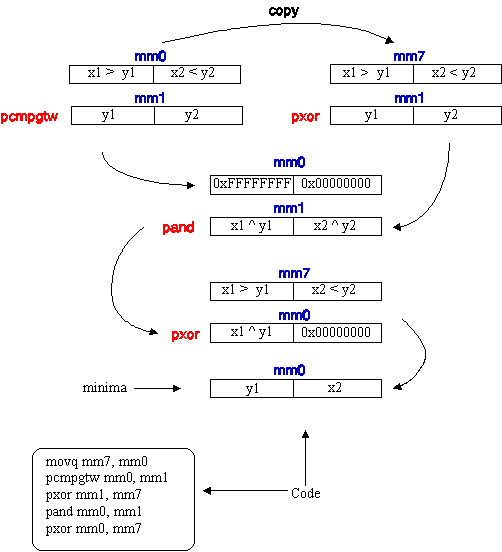### 2.3. Alignment of Operands

It is important for the data structures passed to the viterbi_mmx function to be aligned to 8 byte boundaries. The viterbi_mmx function assumes that the data is already properly aligned. There is a penalty associated with misaligned data which can severely degrade the performance of the function. The C code listing provided in Section 4.0 here does not align the data. Either the alignment can be done during allocation or can be done after allocation by shifting the data structure such that it gets properly aligned. This shifting can be done by a simple routine.

## 3.0. PERFORMANCE GAINS

This section compares the performance of the viterbi_mmx core function with an estimate of the same core implemented using scalar instructions. In computing the performance we assume ideal conditions of all data in cache and no misaligned accesses.

The viterbi_mmx core processes four states every 24 clock cycles. This works out to 6 clocks for every state. Example 4. shows (in pseudo-code) what would be required in order to write the core using scalar instructions to process a single state.

Example 4. Scalar Code (Pseudo-Code)
```core:
1. 	Move Dist(j, t) to Register1
2. 	Move Dist(j-1, t) to Register2
3. 	Move Dist(j-2, t) to Register3
4. 	Add aProb(j, j) to Register1
5. 	Add aProb(j, j-1) to Register2
6. 	Add aProb(j, j-2) to Register3
7. 	Find Minimum of Register1, Register2 and Register3 into Register1
8. 	Add bProb(j, t) to Register1
9. 	Write Register1 to Dist(j, t)
10. 	Decrement nCountReg
```

Here Steps 1 to 3 involve a move instruction with memory operand. Steps 4 to 6 involve an add instruction with a memory operand. Steps 8 and 9 each involve an add instruction and a move instruction respecively, each with a memory operand. Steps 10 and 11 involve pairable instructions. Step 7 involves at least six instructions (two sets of compare, move and test-and-jump instructions). So even in the best case assuming we unroll the loop to process two states at a time we can pair only the six instructions at Step 7. So our instruction count to process a single state for the best scheduled code becomes 3 (steps 1-3) + 3 (steps 4-6) + 3 (step 7) + 2 (steps 8, 9) + 1 (steps 10, 11) + 1 (step 12) i.e. a total of 13 clocks. Note that this is only an estimate and probably represents the best case.

In summary the MMX code performs slightly better than 2X times the scalar code. This can be explained by the fact that the MMX code is manipulating two 32-bit operands in a single clock cycle while the scalar code is manioperating only on a single 32-bit operand in a manipulating a single 32-bit operand in one clock cycle.

## 4.0. CODE LISTINGS

This section contains the assembly and C code listings. The assembly code implements the viterbi_mmx function using MMX instructions. The C code contains a straight C code implementation (viterbi_c) of the same Viterbi function for testing purposes. The main program in the C listing executes and compares the MMX code and C code implementations for a few small data sets (the results for both versions, should, naturally, be the same). The results of the comparisons are printed out to the standard console output.

Example 5 shows the assembly code listing and Example 6 the C code listing.

Example 5. Assembly Code Listing
```	TITLE	viterbi.asm
.486P
.model FLAT

PUBLIC_	_viterbi_mmx

_DATA	SEGMENT
_DATA	ENDS

_TEXT	SEGMENT

_obsVect\$ = 8
_obsLen\$ = 12
_aProb\$ = 16
_bProb\$ = 20
_pi\$ = 24
_nStates\$ = 28
_dist\$ = 32
_obsNo\$ = -4
_nCount\$ = -8
_nRemain\$ = -12

nCountReg	EQU	edx
nCount		EQU	DWORD PTR _nCount\$[ebp]
obsNoReg	EQU	edi
nRemain 	EQU DWORD PTR _nRemain\$[ebp]

_viterbi_mmx	PROC NEAR

push	ebp
mov	ebp, esp
sub esp, 12
push	esi
push	edi

cmp DWORD PTR_obsLen\$[ebp], 0
jle done
cmp DWORD PTR_nStates\$[ebp], 0
jle done

; For each state i, initialize dist[i] = pi[i] + b[obsVect][i]
;
mov esi,_bProb\$[ebp]
mov eax,_obsVect\$[ebp]
mov eax, [eax]
mov  esi, [esi + eax*4]
xor eax, eax
mov ebx,_dist\$[ebp]
mov ecx,_pi\$[ebp]

init:
mov edx, [ecx + eax*4]
mov [ebx + eax*4], edx
inc eax
cmp eax,_nStates\$[ebp]
jne init

mov obsNoReg, 1

; The states are processed four at a time. The number of quads to process
; is saved in nCount and nCountReg. The number of remaining states
; (i.e. 0, 1, 2 or 3) is saved in nRemain.

mov nCountReg,_nStates\$[ebp]
cmp nCountReg, 0
je done
shr nCountReg, 2
mov nCount, nCountReg
mov ebx, nCountReg
shl ebx, 2
mov eax,_nStates\$[ebp]
sub eax, ebx
mov nRemain, eax

mainLoop:
; Main loop for advancing the time index. For each
; time index process four states at time in FourStateLoop:

; Exit if end of observations
;
cmp obsNoReg,_obsLen\$[ebp]
je done

; Move addresses and count register
;
mov nCountReg, nCount

; for the current observation symbol
;
mov eax,_obsVect\$[ebp]
mov eax, [eax + obsNoReg*4]
inc obsNoReg

cmp nCountReg, 0
je checkLastStates

FourStateLoop:
; Core loop to process four states at a time
; for a given time index.

movq mm3, mm1

movq mm7, mm1

pcmpgtd	mm1, mm2

movq mm6, mm0

pxor mm2, mm7

pand mm1, mm2

pxor mm1, mm7

pcmpgtd	mm0, mm1

pxor mm1, mm6
movq mm7, mm4

pcmpgtd	mm4, mm5
pand mm0, mm1

pxor mm5, mm7
pxor mm0, mm6

pand mm4, mm5

pxor mm4, mm7
movq mm6, mm3

pcmpgtd	mm3, mm4

pxor mm4, mm6

pand mm3, mm4

pxor mm3, mm6

dec nCountReg

cmp nCountReg, 0
jz checkLastStates

jmp FourStateLoop

checkLastStates:
; Check the remaining number of states
; and process appropriately

mov nCountReg, nRemain
cmp nCountReg, 1
je lastState
cmp nCountReg, 2
je lastTwoStates
cmp nCountReg, 3
je lastThreeStates
jmp mainLoop

lastThreeStates:
; Three states left to process

movq mm7, mm1

movq mm6, mm0

pcmpgtd	mm1, mm2
pxor mm2, mm7

pand mm1, mm2

pxor mm1, mm7

pcmpgtd	mm0, mm1
pxor mm1, mm6

pand mm0, mm1

pxor mm0, mm6

jmp lastState

lastTwoStates:
; Two states left to process

; Process the last two states
;

; Get the minimum of mm0 and mm2 into mm0

movq mm7, mm0
pcmpgtd	mm0, mm2
pxor mm2, mm7
pand mm0, mm2
pxor mm0, mm7

jmp mainLoop

lastState:
; Only one state left to process

jmp mainLoop
done:
; Find the minimum dist[i] for all states i and return in eax
;
mov ebx, 1
cmp ebx, _nStates\$[ebp]
je exit
minLoop:

jle noSwitch
noSwitch:
inc ebx
cmp ebx, _nStates\$[ebp]
jne minLoop
exit:
pop	edi
pop	esi
pop ebp
ret	0

_viterbi_mmx	ENDP

END
```

Example 6. C Code Listing

```#include <stdio.h>
#include <stdlib.h>

/* HI is a large value used for padding data structures. Effectively this will be
* a "dont care" when taking the minimum in the Viterbi function.
*/
#define HI 0xFFFF

/* Return the minimum of two integers. Note the macro __min can be used
* directly if attention is paid to the parenthesis in the arithmetic
* expressions.
*/
int imin(int a, int b)
{
int i;

i = __min(a, b);

return i;
} /* imin */

/* Function template for the Viterbi MMX function call */
int viterbi_mmx(unsigned int *obsVect, int obsLen, unsigned int *aProb,
unsigned int **bProb, unsigned int *pi, int nStates, int *distBuffer);

/* C implementation for the Viterbi function for discrete HMMs. The arguments are
* described briefly here but described in detail in the accompanying application notes.
* obsVect is the vector of VQ indices corresponding to the continuous observations
* obsLen is the length of the observation
* aProb is the vector of transition probabilities (format described in the appliation notes)
* bProb is the matrix of output probabilities. bProb points to a vector of pointers to row
* vectors. Each row index corresponds to the VQ index and each column index corresponds to
* the state number.
* pi is the vector of initial probabilities.
* nStates is the number of states.
* distBuffer is used by the function for temporary storage of distances.
*/

int viterbi_c(unsigned int *obsVect, int obsLen, unsigned int *aProb,
unsigned int **bProb, unsigned int *pi, int nStates, int *distBuffer)
{
int i, j, minDist;
int *Dist = distBuffer;
unsigned int *b, *aProbTemp = aProb;
int nCount, nRemain;

/* Initialiazation */
for (i = 0; i < nStates; i++) {
Dist[i] = pi[i] + bProb[obsVect][i];
}

/* nCount will contain the number of times to execute the main loop
* which processes four states at a time. nRemain contains the number
* of states remaining after processing four at a time (i.e. 0, 1, 2 or 3)
*/
nCount = nStates >> 2;
nRemain = nStates - (nCount << 2);

/* Iterate through each observation (i.e. increment time index) */
for (i = 1; i < obsLen; i++)
{
b = bProb[obsVect[i]];
Dist = distBuffer;
aProb = aProbTemp;

/* Process four states at a time */
for (j = 0; j < nCount; j++) {
Dist = b + imin(Dist + aProb, imin(Dist + aProb,
Dist + aProb));
Dist = b + imin(Dist + aProb, imin(Dist + aProb,
Dist + aProb));
Dist = b + imin(Dist + aProb, imin(Dist + aProb,
Dist + aProb));
Dist = b + imin(Dist + aProb, imin(Dist + aProb,
Dist + aProb));

/* Update addresses for the nex four states */
Dist = Dist + 4;
b = b + 4;
aProb = aProb + 12;
} /* for */

/* Process the remaining states if any (1, 2 or 3 remaining states) */
switch (nRemain)
{
case 3 :
{
Dist = b + imin(Dist + aProb, imin(Dist + aProb,
Dist + aProb));
Dist = b + imin(Dist + aProb, imin(Dist + aProb,
Dist + aProb));
Dist = b + Dist + aProb;
break;
}
case 2 :
{
Dist = b + imin(Dist + aProb, Dist + aProb);
Dist = b + Dist + aProb;
break;
}
case 1 :
{
Dist = b + Dist + aProb;
break;
}
} /* case */

} /* for */

/* Find the return the minimum distance in distBuffer */
Dist = distBuffer;
minDist = distBuffer;
for (i = 1; i < nStates; i++)
if (Dist[i] < minDist)
minDist = Dist[i];

return minDist;
} /* viterbi_c */

/* Return TRUE if argument is an even number, else FALSE */
int evenP(int n)
{
return (n == ((n >> 1) << 1));
}

#define maxStates 20

#define nSymbols 3

/* Main program to test viterbi_c and viterbi_mmx for small datasets */
void main(int argc, char *argv[]){

unsigned int b0[maxStates], b1[maxStates], b2[maxStates];
unsigned int aProb[3 * maxStates];

unsigned int *bProb[nSymbols] = {b0, b1, b2};
unsigned int pi[maxStates];
unsigned int obsVect[2*maxStates];
int distBuffer[maxStates + 2];
int dist_mmx, dist_c, i;
int nStates, obsLen;

/* Create the datasets and fill in aProb, bProb, pi, and obsVect */
for (nStates = 1; nStates <= maxStates; nStates++)
{

for (i = 0; i < nStates; i++)
{
b0[i] = i + 1;
b1[i] = i + 3;
b2[i] = i + 5;
pi[i] = HI;
} /* for */

pi[nStates - 1] = 0;

obsLen = 2 * nStates;
for (i = 0; i < obsLen; i++)
obsVect[i] = i % nSymbols;

if (evenP(nStates))
{
for (i = 0; i < nStates * 3; i++)
aProb[i] = i + 1;
aProb[nStates*3 - 1] = HI;
aProb[nStates*3 - 2] = HI;
aProb[nStates*3 - 3] = HI;
}
else
{
for (i = 0; i < (nStates - 1)*3; i++)
aProb[i] = i + 1;
aProb[(nStates - 1)*3 - 1] = HI;
aProb[(nStates - 1)*3] = (nStates - 1)*3 + 1;
}

distBuffer[nStates] = HI; distBuffer[nStates + 1] = HI;
dist_mmx = viterbi_mmx(obsVect, obsLen, aProb, bProb, pi, nStates, distBuffer);
distBuffer[nStates] = HI;  distBuffer[nStates + 1] = HI;
dist_c = viterbi_c(obsVect, obsLen, aProb, bProb, pi, nStates, distBuffer);

printf(" nStates = %3d, mmx_dist = %6d, c_dist = %6d \n", nStates, dist_mmx, dist_c);
} /* for */

getchar();

} /* main */

```Legal Stuff © 1997 Intel Corporation